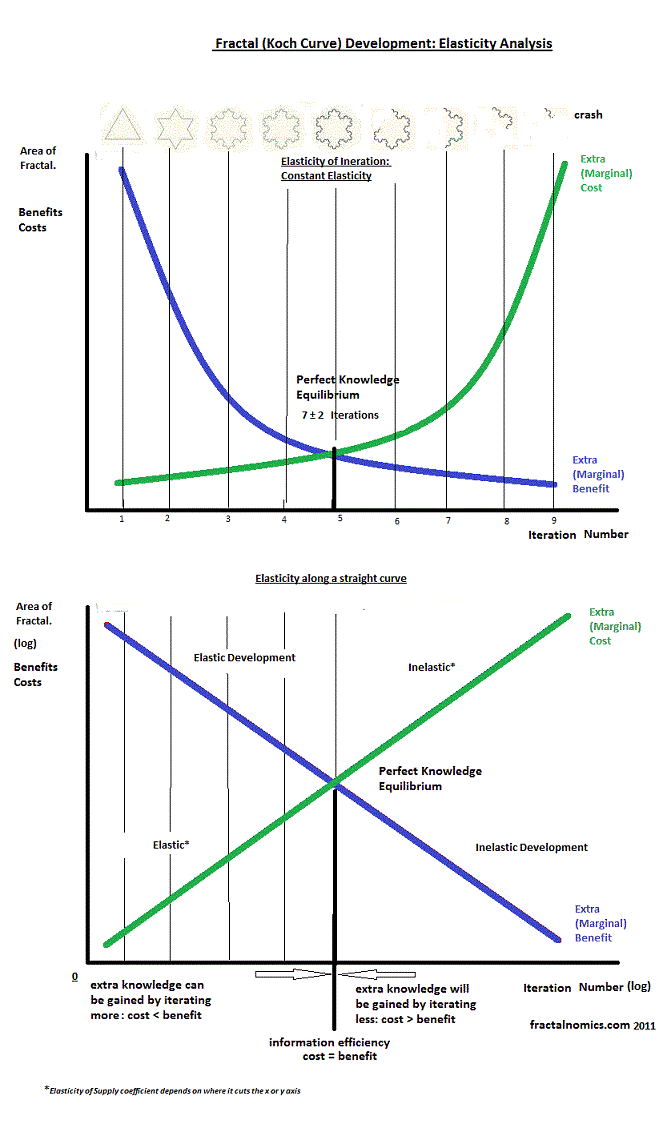## Posts

### Fractal (Information) DecayFractal Decay As shown in the animation below, and described in my earlier entries, the fractal demonstrates development and growth: but if this reversed, it also demonstrates decay. Developing from the  first simple iteration to the complex, and in reverse, decay from the complex to the simple; from the snowflake to the triangle. fractal growth and development Analysis Below are two diagrams that analyse the Koch Snowflake fractal: the top diagram shows the exponential, and the lower the log. Both are split vertically (with a 'black' line of reflection) - showing development on the left side and decay on the right side.  - keep your eye on the snowflake..  The blue curve  shows the extra benefit of another iteration (in terms of Area), and  green - the extra cost of  producing or iterating.  As fractal development is exponential, it follows that so is decay. The above diagram of the two shows the exponential curve - with a constant elasticity (or sens

### The credit card effect

The credit card effect (as opposed to 'the butterfly effect') - one persons (credit card) debt could bankrupt a country - or even the world's economy. This is the perfect economic fractal example where dangerous massive debt burdens have migrated from the small scale (individual) to the large scale (country);  the principle or idea (of debt) is the same, the scale is irrelevant. I explained the world's economic problems to my 10 year old daughter by reducing the problem to her scale - it was very easy. Today - through the mechanism of (moral hazard) bank bail-outs - it is countries that are getting burdened. Where to next? This is the perfect storm. '

### Object: transformation, formation, creationFrom a triangle, to the formation of a snowflake, this is a (universal) demonstration of creation: this is transformation, the creation of an object. Many to make one. Emergence Q. Is the original triangle the big bang? Animation of Koch Snowflake development

### Fractal Elasticity along the straight line curve.Fractal Elasticity - along the straight line curve. Click to see most recent developements that complement this entry. After discovering in my early blog that the elasticity of the Koch Snowflake fractal  is constant, I have since pondered on what is the meaning of all this? Economic theory suggests that all objects have this constant elasticity or are logarithmic in nature. The next thing to to is to straighten out the fractal curves. I produced the following diagrams to do just that and to demonstrate the change in fractal elasticity as the fractal developments. The above diagram shows constant elasticity, and the below variable elasticity along the straight (log) curve.

### (Christmas) tree Lorenz CurveAfter completing my  Lorenz analysis of the Koch Snowflake fractal  I set upon analysing a real life fractal and chose a Christmas tree. This has been a side interest from my core fractal work and thinking so I have not written it up as a 'science report'. I am not sure of the species of tree I selected, but it is typical conifer of Northern hemisphere. Method I trimmed all the branches off the tree, counted them, weighed them, recorded results, then ranked the branches from lightest to heaviest; completed a cumulative percentage rank of weight and count table, and finally graphed the results. Branches everywhere: Below is the Christmas tree Lorenz Curve in terms of weight. Note that 'cumulative percentage of triangle weight' should read branch rather than triangle. I found that there were 5 levels of branches. I will be honest with you, I did not continue counting and weighing the branches in detail after the 3 level - the time cost was just so high and it

### 1 + 1 does not equal 2

It came to me yesterday - in an epiphany: 1 + 1 does not equal 2 : if it does it is only half the answer, the other half lies in understanding chaos and fractals. The definition of (or insight from) the fractal is: same but different (or regular irregularity) - at all scales. Fractals show us how no one object is the same, they are complex, they are different . The 'same ' component of the definition is quantitative, and met or described as the 1 + 1. The different is qualitative and describes (at least) the diversity, complexity or unpredictability of the object. Update 2015 I have long thought about the my early entry and now know more. If 2 identical objects are added together, they equal 1. They are indistinguishable. I have also leant this is an assumption at the quantum level where particles are assumed to be identical; more support to my fractal quantum theory. I plan to write all this up in one paper as soon as I can. Blair

### Rationality and Chaos

Updated: 29th Nov. 2012 This is an entry I have been wanting to do for some time, and is the first of three on fractal insights I have discovered on the economic assumptions (rationality, ceteris paribus, and perfect knowledge). This is a very difficult subject to describe, I hope I give it justice. I strongly believe that the issue of understanding of rationality is closely related to - if not the same as - that of understanding 'chaos': that is to say, complex systems are unpredictable. If we are to understand rationality, then should understand chaos, and thus, fractals. The definition of the fractal (attractor) is: same but different , at all scales. In our Economic models we use the assumption ceteris paribus: we hold all other variables constant,  and treat all persons as rational, so as to see the order (or the 'same', as in the definition), amongst complexity -  just as other science's do.  This definition maybe adapted or interpreted in this context